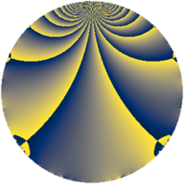# Properties

 Label 2001.2.hLevel $2001$ Weight $2$ Character orbit 2001.h Rep. character $\chi_{2001}(2000,\cdot)$ Character field $\Q$ Dimension $236$ Sturm bound $480$

# Related objects

## Defining parameters

 Level: $$N$$ $$=$$ $$2001 = 3 \cdot 23 \cdot 29$$ Weight: $$k$$ $$=$$ $$2$$ Character orbit: $$[\chi]$$ $$=$$ 2001.h (of order $$2$$ and degree $$1$$) Character conductor: $$\operatorname{cond}(\chi)$$ $$=$$ $$2001$$ Character field: $$\Q$$ Sturm bound: $$480$$

## Dimensions

The following table gives the dimensions of various subspaces of $$M_{2}(2001, [\chi])$$.

Total New Old
Modular forms 244 244 0
Cusp forms 236 236 0
Eisenstein series 8 8 0

## Trace form

 $$236q + 224q^{4} - 14q^{6} - 12q^{9} + O(q^{10})$$ $$236q + 224q^{4} - 14q^{6} - 12q^{9} - 16q^{13} + 232q^{16} - 52q^{24} + 220q^{25} - 58q^{36} - 252q^{49} - 156q^{52} + 28q^{54} + 62q^{58} + 180q^{64} - 106q^{78} - 100q^{81} - 60q^{82} - 36q^{93} + 76q^{94} - 54q^{96} + O(q^{100})$$

## Decomposition of $$S_{2}^{\mathrm{new}}(2001, [\chi])$$ into newform subspaces

The newforms in this space have not yet been added to the LMFDB.